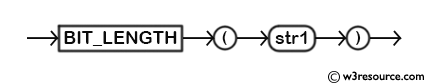# MySQL BIT_LENGTH() function

## BIT_LENGTH() function

MySQL BIT_LENGTH() returns the length of the given string in bits.

This function is useful in -

• It allows us to determine string length in bits rather than bytes.
• The function can help estimate storage requirements for a particular string.
• The function is often used with other bitwise operations or functions.

Syntax:

```BIT_LENGTH (str1)
```

Argument:

Name Description
str1 A string whose BIT_LENGTH value is to be retrieved.

Syntax Diagram:MySQL Version: 8.0

Example: MySQL BIT_LENGTH() function

The following MySQL statement will return the length of the given string 'my text' in bits, i.e. 56.

Code:

``````SELECT BIT_LENGTH('my text');
```
```

Output:

```mysql> SELECT BIT_LENGTH('my text');
+-----------------------+
| BIT_LENGTH('my text') |
+-----------------------+
|                    56 |
+-----------------------+
1 row in set (0.02 sec)
```

Example of MySQL BIT_LENGTH() function using table

The following MySQL statement will return the length of each city mentioned in pub_city column in bits.

Code:

``````SELECT pub_city,
BIT_LENGTH(pub_city)  AS 'bit length'
FROM publisher;
```
```

Sample table: publisher

Output:

```mysql> SELECT pub_city,
-> BIT_LENGTH(pub_city)  AS 'bit length'
-> FROM publisher;
+-----------+------------+
| pub_city  | bit length |
+-----------+------------+
| New York  |         64 |
| Mumbai    |         48 |
| London    |         48 |
| Houstan   |         56 |
| New York  |         64 |
| Cambridge |         72 |
| New Delhi |         72 |
+-----------+------------+
8 rows in set (0.02 sec)
```

Use the BIT_LENGTH() function with string concatenation

To get the bit length of a concatenated string the following MySQL statement can be used.

Code:

``````SELECT BIT_LENGTH(CONCAT('Hello ', ' World'));
```
```

Output:

```BIT_LENGTH(CONCAT('Hello ', ' World'))|
--------------------------------------+
96|
```

The concatenated string 'HelloWorld' has a length of 10 characters, so the total bit length is 10 * 8 = 80. Additionally, there are 16 bits for the 2 spaces between 'Hello' and 'World', resulting in a total bit length of 96.

Video Presentation:

All String Functions (Slides presentation)

Previous: BIN
Next: CHAR_LENGTH

﻿